Vectors - Motion and Forces in Two Dimensions - Lesson 3 - Forces in Two Dimensions

In Unit 2 we studied the use of Newton's second law and free-body diagrams to determine the net force and acceleration of objects. In that unit, the forces acting upon objects were always directed in one dimension. There may have been both horizontal and vertical forces acting upon objects; yet there were never individual forces that were directed both horizontally and vertically. Furthermore, when a free-body diagram analysis was performed, the net force was either horizontal or vertical; the net force (and corresponding acceleration) was never both horizontal and vertical. Now times have changed and you are ready for situations involving forces in two dimensions. In this unit, we will examine the effect of forces acting at angles to the horizontal, such that the force has an influence in two dimensions - horizontally and vertically. For such situations, Newton's second law applies as it always did for situations involving one-dimensional net forces. However, to use Newton's laws, common vector operations such as vector addition and vector resolution will have to be applied. In this part of Lesson 3, the rules for adding vectors will be reviewed and applied to the addition of force vectors.

### Analyzing Three Forces to Determine the Net Force

Methods of adding vectors were discussed earlier in Lesson 1 of this unit. During that discussion, the head to tail method of vector addition was introduced as a useful method of adding vectors that are not at right angles to each other. Now we will see how that method applies to situations involving the addition of force vectors.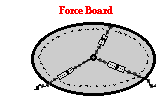A force board (or force table) is a common physics lab apparatus that has three (or more) chains or cables attached to a center ring. The chains or cables exert forces upon the center ring in three different directions. Typically the experimenter adjusts the direction of the three forces, makes measurements of the amount of force in each direction, and determines the vector sum of three forces. Forces perpendicular to the plane of the force board are typically ignored in the analysis.

Suppose that a force board or a force table is used such that there are three forces acting upon an object. (The object is the ring in the center of the force board or force table.) In this situation, two of the forces are acting in two-dimensions. A top view of these three forces could be represented by the following diagram.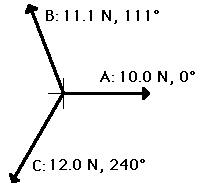The goal of a force analysis is to determine the net force and the corresponding acceleration. The net force is the vector sum of all the forces. That is, the net force is the resultant of all the forces; it is the result of adding all the forces together as vectors. For the situation of the three forces on the force board, the net force is the sum of force vectors A + B + C.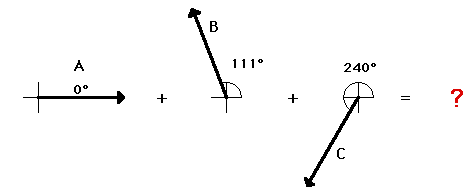One method of determining the vector sum of these three forces (i.e., the net force") is to employ the method of head-to-tail addition. In this method, an accurately drawn scaled diagram is used and each individual vector is drawn to scale. Where the head of one vector ends, the tail of the next vector begins. Once all vectors are added, the resultant (i.e., the vector sum) can be determined by drawing a vector from the tail of the first vector to the head of the last vector. This procedure is shown below. The three vectors are added using the head-to-tail method. Incidentally, the vector sum of the three vectors is 0 Newton - the three vectors add up to 0 Newton. The last vector ends where the first vector began such that there is no resultant vector.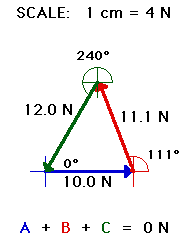The purpose of adding force vectors is to determine the net force acting upon an object. In the above case, the net force (vector sum of all the forces) is 0 Newton. This would be expected for the situation since the object (the ring in the center of the force table) is at rest and staying at rest. We would say that the object is at equilibrium. Any object upon which all the forces are balanced (Fnet = 0 N) is said to be at equilibrium.

Quite obviously, the net force is not always 0 Newton. In fact, whenever objects are accelerating, the forces will not balance and the net force will be nonzero. This is consistent with Newton's first law of motion. For example consider the situation described below.

### An Example to Test Your UnderstandingA pack of five Artic wolves are exerting five different forces upon the carcass of a 500-kg dead polar bear. A top view showing the magnitude and direction of each of the five individual forces is shown in the diagram at the right. The counterclockwise convention is used to indicate the direction of each force vector. Remember that this is a top view of the situation and as such does not depict the gravitational and normal forces (since they would be perpendicular to the plane of your computer monitor); it can be assumed that the gravitational and normal forces balance each other. Use a scaled vector diagram to determine the net force acting upon the polar bear. Then compute the acceleration of the polar bear (both magnitude and direction). When finished, check your answer by clicking the button and then view the solution to the problem by analyzing the diagrams shown below.

The task of determining the vector sum of all the forces for the polar bear problem involves constructing an accurately drawn scaled vector diagram in which all five forces are added head-to-tail. The following five forces must be added.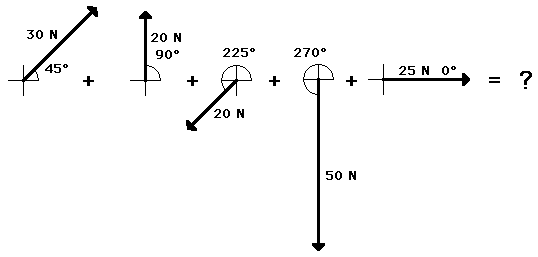The scaled vector diagram for this problem would look like the following: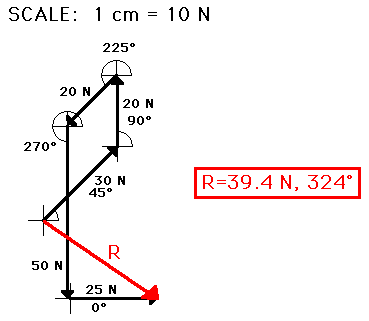The above two problems (the force table problem and the polar bear problem) illustrate the use of the head-to-tail method for determining the vector sum of all the forces. The resultants in each of the above diagrams represent the net force acting upon the object. This net force is related to the acceleration of the object. Thus, to put the contents of this page in perspective with other material studied in this course, vector addition methods can be utilized to determine the sum of all the forces acting upon an object and subsequently the acceleration of that object. And the acceleration of an object can be combined with kinematic equations to determine motion information (i.e., the final velocity, the distance traveled, etc.) for a given object.

### Sometimes 10 + 10 = 10

In addition to knowing graphical methods of adding the forces acting upon an object, it is also important to have a conceptual grasp of the principles of adding forces. Let's begin by considering the addition of two forces, both having a magnitude of 10 Newton. Suppose the question is posed:

10 Newton + 10 Newton = ???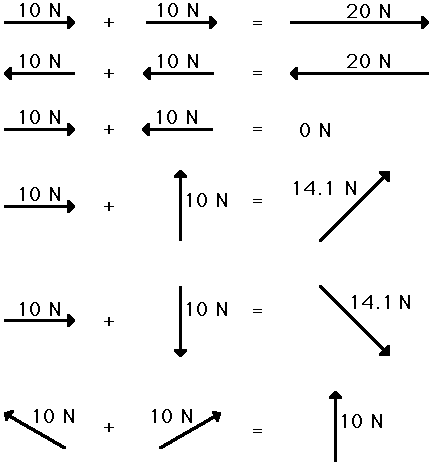The above diagram shows what is occasionally a difficult concept to believe. Many students find it difficult to see how 10 N + 10 N could ever be equal to 10 N. For reasons to be discussed in the next section of this lesson, 10 N + 10 N would equal 10 N whenever the two forces to be added are at 30 degrees to the horizontal. For now, it ought to be sufficient to merely show a simple vector addition diagram for the addition of the two forces (see diagram below).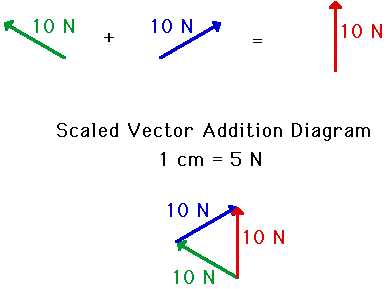### We Would Like to Suggest ...Sometimes it isn't enough to just read about it. You have to interact with it! And that's exactly what you do when you use one of The Physics Classroom's Interactives. We would like to suggest that you combine the reading of this page with the use of our Name That Vector Interactive, our Vector Addition Interactive, or our Vector Guessing Game Interactive. All three Interactives can be found in the Physics Interactive section of our website and provide an interactive experience with the skill of adding vectors.

Answer the following questions and then view the answers by clicking on the button.

1. Barb Dwyer recently submitted her vector addition homework assignment. As seen below, Barb added two vectors and drew the resultant. However, Barb Dwyer failed to label the resultant on the diagram. For each case, that is the resultant (A, B, or C)? Explain.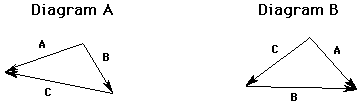2. Consider the following five force vectors.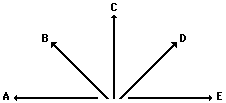Sketch the following and draw the resultant (R). Do not draw a scaled vector diagram; merely make a sketch. Label each vector. Clearly label the resultant (R).

A + C + D

B + E + D

3. On two different occasions during a high school soccer game, the ball was kicked simultaneously by players on opposing teams. In which case (Case 1 or Case 2) does the ball undergo the greatest acceleration? Explain your answer.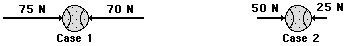4. Billie Budten and Mia Neezhirt are having an intense argument at the lunch table. They are adding two force vectors together to determine the resultant force. The magnitude of the two forces are 3 N and 4 N. Billie is arguing that the sum of the two forces is 7 N. Mia argues that the two forces add together to equal 5 N. Who is right? Explain.

5. Matt Erznott entered the classroom for his physics class. He quickly became amazed by the remains of some of teacher's whiteboard scribblings. Evidently, the teacher had taught his class on that day that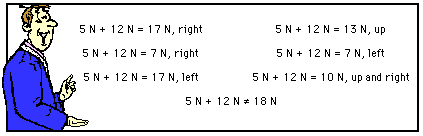Explain why the equalities are indeed equalities and the inequality must definitely be an inequality.

Next Section: